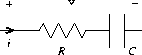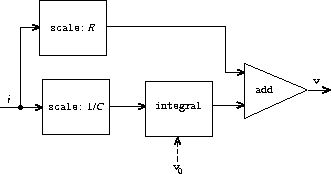# An RC circuitFigure 3.33: An RC circuit and the associated signal-flow diagram.

We can model electrical circuits using streams to represent the values of currents or voltages at a sequence of times. For instance, suppose we have an `RC` circuit consisting of a resistor of resistance `R` and a capacitor of capacitance `C` in series. The voltage response `v` of the circuit to an injected current `i` is determined by the formula in figure 3.33, whose structure is shown by the accompanying signal-flow diagram.

Write a procedure `RC` that models this circuit. `RC` should take as inputs the values of `R` , `C` , and `dt` and should return a procedure that takes as inputs a stream representing the current `i` and an initial value for the capacitor voltage `v₀` and produces as output the stream of voltages `v` . For example, you should be able to use `RC` to model an `RC` circuit with `R = 5` ohms, `C = 1` farad, and a `0.5` -second time step by evaluating `(define RC1 (RC 5 1 0.5))` . This defines `RC1` as a procedure that takes a stream representing the time sequence of currents and an initial capacitor voltage and produces the output stream of voltages.